Latest Banking jobs   »   Reasoning Ability Quiz For SBI Clerk...

# Reasoning Ability Quiz For SBI Clerk Prelims 2021- 8th June

Directions (1-5): Study the following information to answer the given questions.
Eight students are sitting around a square table in such a way four of them sit at four corners while four sits in the middle of each of the four sides. The one who sit at the corners face the centre and others facing outside. U face the centre who is third to the left of V. S sits at one of the middle sides of the table and U is not the neighbor of S. Q sits third to the right of P who is not an immediate neighbor of T. Q sits second to the right of T. R does not face the centre. W does not sit to immediate left of P.

Q1. Who among the following sits third to the left of W?
(a) U
(b) R
(c) T
(d) S
(e) None of these

Q2. Who among the following faces Q?
(a) P
(b) U
(c) W
(d) T
(e) None of these

Q3. How many persons sit between V and T, when counted right of V?
(a) One
(b) Four
(c) Two
(d) Three
(e) None

Q4. Which of the following statement is true about R?
(a) U sits immediate left of R
(b) S sits second to the left of R
(c) Only one person sit between R and Q
(d) R sits opposite to S
(e) None is true

Q5. What is the position of P with respect to S?
(a) Third to the left
(b) Second to the left
(c) Immediate right
(d) Fourth to the right
(e) None of these

Directions (6-7): Study the following information carefully and answer the given questions:
A, B, C, D, E, F, G, H, I and J are ten members of the family. A is married to J. B is daughter of I. J and I are brothers. E is sister of B. F is mother-in-law of A. I is the son of G. C is the mother of E. D is the son of C. H is the mother-in-law of J.

Q6.How is J related to E?
(a) Uncle
(b)Aunt
(c) Father
(d) Mother
(e) Brother

Q12.How is G related to B?
(a) Grandfather
(b) Sister
(c) Grandmother
(d) Mother
(e) Father

Directions (8-10): Study the following information carefully to answer the given questions:
There are eight members namely A, B, C, D, E, F, G and H are in a family in which three married couple and three generation. B is sister of A. D is brother of G. D has only one son. F is the wife of D. F is the daughter of B. A is brother-in-law of H. I is daughter in law of F.

Q8. How many male members in a family?
(a) Five
(b) More than Five
(c) Three
(d) Four
(e) Can’t be determine

Q9. Who among the following is Father-in-law of I?
(a) D
(b) B
(c) G
(d) F
(e) None of these

Q10. How C is related to G?
(a) Father
(b) Nephew
(c) Daughter
(d) Niece
(e) None of these

Q11. The position of how many alphabets will remain unchanged if each of the alphabets in the word ‘Organization’ is arranged in alphabetical order from left to right?
(a) Two
(b) Three
(c) One
(d) Four
(e) Five

Q12.Which of the following elements should come in a place ‘?’ ?
AC24 EG20 JL15 ?
(a) OQ10
(b) PR9
(c) NP11
(d) MQ10
(e) None of these

Q13. In a class of 40 students Rohan rank is 21 from the bottom and Arun is 2 rank below Rohan, then what is the rank of Arun from the top?
(a) 21
(b) 22
(c) 25
(d) 24
(e) 20

Q14. How many pairs of letters are there in the word “SUPERNATURAL” each of which have as many letters between them in the word as they have between them in the English alphabetical series?
(a) Five
(b) Three
(c) Two
(d) Four
(e) More than five

Q15. Which of the following will be definitely false, if the given expression
“M=Z>S =L<K≥Q=D>C >W=O> I” is definitely true?
(a) L < M
(b) K > S
(c) O < Q
(d) K ≥ C
(e) I < D

Practice More Questions of Reasoning for Competitive Exams:

###### SBI Clerk Study Plan 2021

Solutions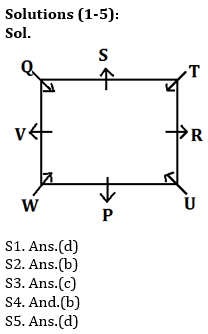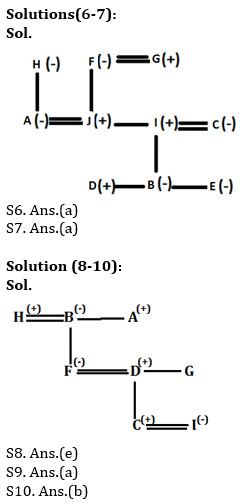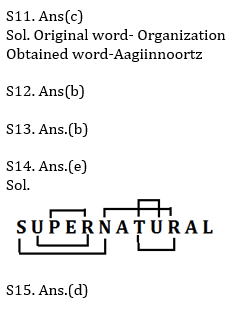#### Congratulations!

Incorrect details? Fill the form again here

•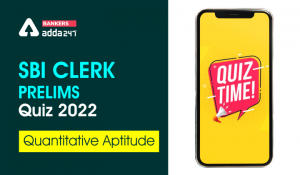Quantitative Aptitude Quiz For SBI Clerk...
•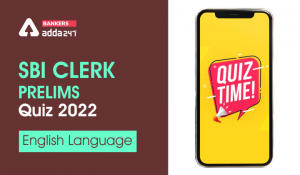English Quizzes For SBI Clerk Prelims 20...
•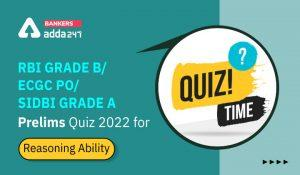Reasoning Ability Quiz For RBI Grade B/ ...
•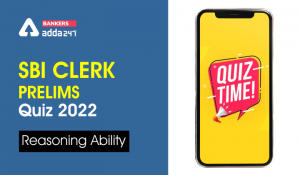Reasoning Ability Quiz For SBI Clerk Pre...
•Quantitative Aptitude Quiz For SBI Clerk...
•Quantitative Aptitude Quiz For SBI Clerk...